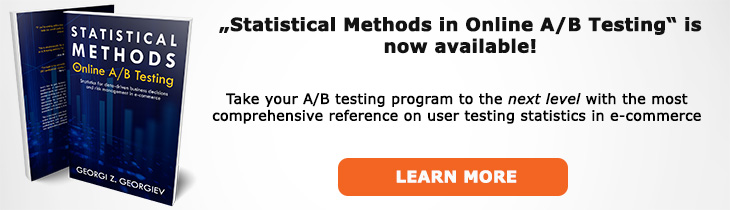# What does "Estimation" mean?

Definition of Estimation in the context of A/B testing (online controlled experiments).

## What is Estimation?

Estimation is the use of statistical methods to establish the true value of a parameter of interest, e.g. the conversion rate or purchase rate of a website, as well as to ascertain the uncertainty related to the estimation procedure. A prime example of estimation in A/B testing is the use of procedures for constructing confidence interval and Maximum-Likelihood Estimate. A good estimator is expected to be finite-sample unbiased, fully-efficient and sufficient, while also being asymptotically consistent.

Unlike hypothesis testing, estimation does not require the specification of a null hypothesis and an alternative hypothesis, however it can easily be translated into a Null Hypothesis Statistical Test due to the mathematical duality between the two approaches. Simply put, a hypothesis defined in a such way so that an XX% confidence interval excludes all its values can be rejected at least at significance level (100-XX)/100.

Issues that come up with hypothesis testing are not foreign to estimations as well. For example, a confidence interval will be affected by unaccounted peeking the same as a statistical test. The choice of what confidence level is appropriate for a given task is also the exact same task as defining what significance threshold to use for a hypothesis test. Violations of the statistical premises behind an estimator also have the same negative effects.

Like this glossary entry? For an in-depth and comprehensive reading on A/B testing stats, check out the book "Statistical Methods in Online A/B Testing" by the author of this glossary, Georgi Georgiev.## Glossary Index by Letter

Select a letter to see all A/B testing terms starting with that letter or visit the Glossary homepage to see all.